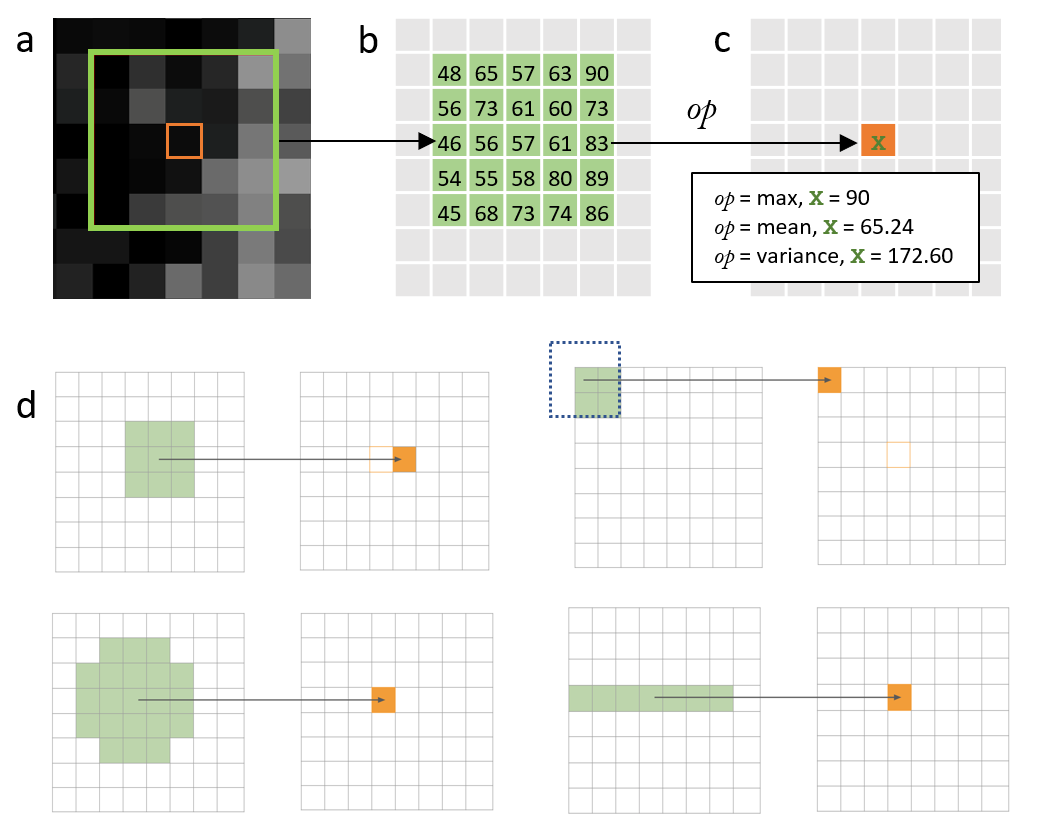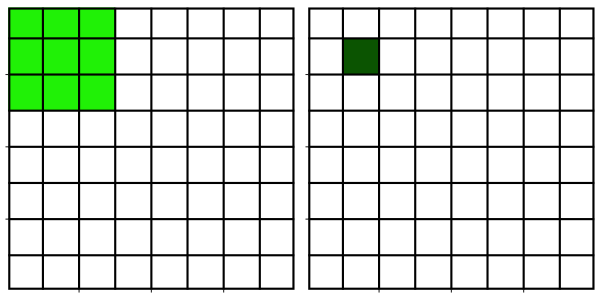## Prerequisites

Before starting this lesson, you should be familiar with:

## Learning Objectives

After completing this lesson, learners should be able to:
• Understand the basic principle of a neighborhood filter.

• Apply basic neighborhood filters to an image.

## Motivation

Images are quite often noisy or have other issues that make them hard to segment, e.g. by means of a simple intensity threshold. Neighborhood filters are often used to enhance the images in order to facilitate better performance of segmentation algorithms.

## Concept map

graph TB P(Pixel coordinate) -->NBH(Neighborhood pixels) SE(Structuring element) --> NBH NBH -->A(Mathematical formula) A -->NP(New pixel value)

## FigureImage filtering with a pixel neighborhood. (a) Raw intensity image with pixel neighborhood (structuring element (SE), outer green box) and central pixel (inner orange box) on which the filtering operation will be performed. (b) Pixel values in the neighborhood. (c) X is the value that would be replaced after operation (indicated as op). Here, max, mean and variance operations are used. Note - One has to carefully look at the data type of the image as some operations can produce large/floating point values. (d) Different SEs (neighborhood in green and affected pixel in orange) top left - SE completely inside image boundaries; top right - SE at image boundaries (padding needed); bottom left - SE with different shape; bottom right - Line SE.

## Activities

#### Mean filter and structuring element exploration

• Explore the effect of a mean filter with different radii
• Investigate the shapes of structuring elements
• Sometimes you would like to know how the SE (the neighborhood) of a filter looks like.
• One way to do this is to apply a maximum filter to a single white pixel and look at the resulting form.
• Example image: xy_8bit_binary__one_foreground_pixel.tif

Show activity for:

## ImageJ GUI

• Mean filter
• Open xy_8bit__nuclei_very_noisy.tif
• It is helpful to first duplicate the image: [Image > Duplicate…] or [Ctrl-Shift-D]
• `Title = mean_1`
• Apply a mean filter to the image such that you can binarize the image into exactly three disjoint foreground regions (the three nuclei). The resulting mask should look like xy_8bit_binary__nuclei_very_noisy.tif
• [Process > Filters > Mean…]
• `Radius = 1 pixels`
• [Image > Adjust > Threshold…]
• `Dark Background`
• `Lower threshold level = 31`
• `Higher threshold level = 255`
• Press `Set`
• Press `Apply`
• [Image > Lookup Tables > Invert LUT]
• Repeat the procedure with radii = 2, 5, 7
• What is the smallest size for the mean filter that does the job?
• `Radius = 2 pixels` and `Lower threshold level = 30` (other values can also be chosen) can remove the noise and find the appropriate nuclei size after manual thresholding
• What happens if the filter size increases?
• The boundaries of segmented nuclei are smoother
• Structuring element inspection

## skimage napari

#### Variance filtering

Show activity for:

## Exercise mean filter

• Open image xy_8bit__noisy_two_nuclei_close.tif
• The aim is to segment the image into foreground/background in order to get a similar result to xy_8bit_binary__noisy_two_nuclei_close.tif
• To achive this aim, try thresholding the image after applying a mean filter with different radii.
• Try thresholding
• Without a mean filter
• After applying a mean filter of radius: 1, 2, 3, or 5
• For each filter setting find the lowest threshold that yields exactly four connected components (the nuclei).
• Is this even possible without applying a mean filter?
• For radius 5, what happens to the size of the segmented nuclei?

## Solution

• [ Image › Duplicate… ]
• [ Process › Filters › Mean… ]
• [ Image > Adjust > Threshold… ]
• Radius 1: threshold = 38
• Radius 2: threshold = 43
• Radius 3: threshold = 48
• Radius 5: threshold = 57
• Only segmenting the four nuclei in unfiltered image does not work, as it yields a large number of undesired connected components (noise).
• Radius 1 yields a reasonable segmentation.
• For radius 5 one has to choose a rather high threshold to still separate some of the nuclei and thus the segmented nuclei become very small.

## Exercise variance filter

• Open image xy_16bit__embryo_transmission.tif
• Apply a variance filter [ Process > Filter > Variance… ] to segment the cell regions from the background
• Hints:
• Convert image to float, because the filter may yield high values [ Image > Type > 32-bit ]
• What filter radius and threshold yield a good segmentation?

## Solution

• [ Image › Rename…]
• Title = input
• [ Image › Duplicate… ]
• Title = variance_5
• [ Image > Type > 32-bit ]
• In variance calculation, pixel values can exceed 255 which is the maximum value that can be achieved in current bit depth (unsigned 8-bit) of input image.
• [ Process › Filters › Variance… ]
• `Radius = 15 pixels`
• [ Image › Adjust › Threshold… ]
• `([x] Dark Background)`
• `Lower threshold level = 1.5`
• `Higher threshold level = 1e30`
• Press `Set`
• Press `Apply`
• Press `Convert to Mask`

## ImageJ Macro

// File > Close All run(“Close All”); // File > Open… open(“C:/Users/akhan/training-resources/image_data/xy_8bit__em_mitochondria_er.tif”);

// Image › Duplicate… run(“Duplicate…”, “title=variance_5”);

// Image > Type > 16-bit (since in variance calculation values can exceed 255 which is current bit depth of input image) run(“16-bit”);

// Process › Filters › Variance… run(“Variance…”, “radius=5”);

// Image › Adjust › Threshold… ([x] Dark Background) setAutoThreshold(“Default dark”);

//Press `Set` button (example upper and lower threshold values are (113, 255)) setThreshold(113, 255);

// Press `Apply` button run(“Convert to Mask”);

// Image › Lookup Tables › InvertLUT… (if highest values are darker and lowest brighter) run(“Invert LUT”);

## Assessment

#### Fill in the blanks

Fill in the blanks, using these words: decrease, increase, size, structuring element, large

• A synonym for neighborhood is __
• The filter radius characterize the filter ___
• ___ filter size can cause a loss of details in the filtered image
• Filter can be used to __ the noise in an image
• The usage of filters can __ the quality of image segmentation/binarization

## Solution

• A synonym for neighborhood is structuring element (SE)
• The filter radius characterize the filter size
• large filter size can cause a loss of details in the filtered image
• Filter can be used to decrease the noise in an image
• The usage of filters can increase the quality of image segmentation/binarization

## Explanations

Neighborhood filters comprise two ingredients: a definition of the pixel neighborhood (size and shape) and a mathematical recipe what to compute on this neighborhood. The result of this computation will be used to replace the value of the central pixel in the neighborhood. This procedure can be applied to several (all) pixels of an image to obtain a filtered image. The animation shows a square neighborhood (3x3) applied to the inner pixels of the image.

There are tons of different neighborhood filters, and you can also invent one!#### The neighborhoods

The neighborhood of a pixel is also called a structuring element (SE) and can have various sizes and shapes. Here, we use one of the simplest and most widely used neighborhoods, namely a circular neighborhood, which is defined by a certain radius. We will explore other shapes of SEs in more detail in a dedicated module.

Since the filtering operation takes into account all the directions of extent of SE, border pixels would be affected in a different way and one has to decide that which values they should assume. Padding is the operation of adding an additional layer around your data to get more accurate values after filtering process. It also gives you option to retain same dimensions for your data after filtering. Common padding methods are using zeros or to mirror/replicate the border pixel values.

#### The math

There are many ways how to cleverly compute on a pixel neighborhood. For example, one class of computations is called convolutional filters, another is called rank filters. Here, we focus on the relatively simple mean and variance filters.

#### Best practice

As usual, everything depends one the scientific question, but maybe one could say to use a filter that changes the image as little as possible.

## Follow-up material

Recommended follow-up modules: